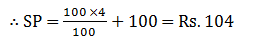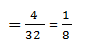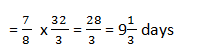# Data Sufficiency Test for IBPS Clerk Mains ExamDirections  (1-5): Each of the questions below consists of a question and three statements numbered A, B and C given below it. You have to decide whether the data provided in the statements are sufficient to answer the questions. Read all the statements and given an answer:

1. What is the cost of painting two adjacent walls of a room which have no window or door?
A. The area of room is 664m².
B. The breadth, height and the length of the room are in the ratio of 4: 5: 7
C. The cost of painting per m² is rs. 50.
1. Both A and B
2. All A, B and C
3. Only C
4. Both B and C
5. Only B

2. How much profit did Naman earn on the cost price of an article by selling it?
A. He got 10% discount on the marked price at the time of purchase.
B. He sold it for Rs. 3120.
C. He earned 4% profit on the marked price.
1. All A, B and C
2. Only A
3. Only B
4. Both A and B
5. Only C

3. What is the present population of the city?
A. The population of the city was 1690000, two years ago.
B. The population of the city increases at the rate of 25% per year.
C. The population of the city decreases by 1/25 of its total population per year.
1. Only C
2. Both A and B
3. All A, B and C
4. Only A
5. Both B and C

4. In how many days will the work be completed by Aryan and Ankush?
A. Aryan is twice as efficient as Ankush.
B. Ankush can complete the work in 32 days.
C. Aryan joins the work after 4 days of the start of work.
1. Only A
2. Only B
3. Both A and B
4. Both B and C
5. All A, B and C

5. What is the area of the hte rectangular field?
A. The perimeter of the field is 240 metres.
B. The width is 10 meters less than the length.
C. The ratio of the length to breadth is 13: 11
1. Both A and B
2. Both B and C
3. Only A
4. Only B
5. Any two of the three

1. 2;
From A, B and C, we get
Area of the room = 664
⇒ 2 (20x² + 28x² + 35x²) = 664
⇒ 116x² = 664
∴ x = 2
∴ Cost of painting
= (Ih + bh) x 50
= (14 + 8  ) x 10 x 50
= Rs. 11000

2. 1;
Let the marked price be Rs. 100
From A, B and C, we get
Cost Price = Rs. 90
By selling the article for Rs. 3120, he gained 4% on the marked price.∴ Sp is 104, when MP  is Rs. 100
SP is 3120, when MP = 100/104 x 3120 = Rs. 3000
∴ Cost price = 3000 x 90/100 = Rs. 2700
So we need all three statements to answer the question.

3. 3;
From A, B and C:
Population of the city two years ago = 1,69,0000
Rate of increase = 25% per year
Rate of decrease = 1/25 x 100 = 4% per year
Hence, total growth of population = 25 - 4 = 31%
So, present population = 1690000 (1+21/100)²
= 2474329
Hence, all the statements are necessary.

4. 5;
From Statement A; Ratio of efficiency of Aryan to that of Ankush = 2: 1
Ratio of time taken by Aryan to that of Ankush = 1:2
From A, B and C;
If Ankush can complete the work in 32 days.
Then, 4 days work of AnkushSo, the remaining workAfter 4 days, Aryan and Ankush both are working together.
Hence 1 day's work of Ankush and AryanNumber of days required to complete the workTotal number of daysSo,all the three statements are required.

5. 5;
From A; 2(I + b) = 240 ⇒ I + b = 120
From B; I = (b + 10) ⇒ I - b = 10These are three equations are of length and breadth. We may solve them by making pair of any two.
∴ Any two of the three will give answer.